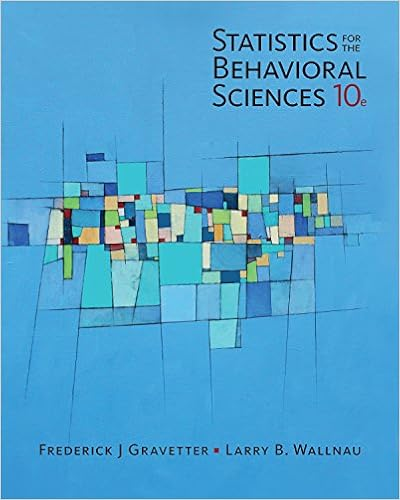# This is incorrect since the population distribution

• Homework Help
• 10

This preview shows page 3 - 6 out of 10 pages.

##### We have textbook solutions for you!
The document you are viewing contains questions related to this textbook.The document you are viewing contains questions related to this textbook.
Chapter 3 / Exercise 1
Statistics for the Behavioral Sciences
Gravetter/WallnauExpert Verified
This is incorrect since the population distribution will not change but rather the sampling distribution is the one which becomes closer to a normal distribution. D P(at least 2) = 1 P(at most 1) = 1 0.652 = 0.348
##### We have textbook solutions for you!
The document you are viewing contains questions related to this textbook.The document you are viewing contains questions related to this textbook.
Chapter 3 / Exercise 1
Statistics for the Behavioral Sciences
Gravetter/WallnauExpert Verified
__________ 10. Suppose that A and B are mutually exclusive and P(A) = 0.3 and P(B) = 0.4. What is P(A B)?
___________ 12. For a set of values, suppose the mean is 10 and the standard deviation is 2. If each value is multiplied by 9 and 4 is then added to each value, what will be the mean and standard deviation for this new set of values? A)Mean 90; standard deviation 6 D) Mean 94; standard deviation 22 B)Mean 90; standard deviation 18 E) Mean 126; standard deviation 22 C)Mean 94; standard deviation 18 F) None of the above New = 9 Old + 4 The mean will be E[New] = 9 E[Old] + 4 = 9(10)+4 = 90. The variance is Var[New] = 92Var[Old] = 92(2)2= 324, which means the standard deviation is 18.
II. BRIEF EXPLANATION. Please answer this question concisely. Do not exceed 6 sentences. Without formulas, equations, or diagrams, briefly explain in your own wordswhat the k-NN algorithm does and how it generally works. (6 pts) Using the formulas: New = 9 Old + 4 The mean will be E[New] = 9 E[Old] + 4 = 9(10)+4 = 90. The variance is Var[New] = 92Var[Old] = 92(2)2= 324, which means the standard deviation is 18. The k-NN algorithm is a classification algorithm that provides a prediction of which class or type a point is. The manner by which it does the classification is looking at a specific number of neighboring points in a training data set and uses a majority rule to predict the class that the point belongs to. For example, if the five nearest neighbors are three Type A points and two are Type B points, then the prediction for the new point will be Type A since more Type A neighbors are in the vicinity of this new point. The rationale here is that the new point is likely going to be similar to the points around it.
NAME: TYPE YOUR NAME HERE OPIM 550 EP: MANAGERIAL STATISTICS Fall 2017 V. R. JOSE MIDTERM PART B ANSWER SHEET INSTRUCTIONS: Please write the number of the question you are answering. Include solutions if
•••